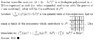# Coefficient of a term

• chaoseverlasting

## Homework Statement

What is the coeff of $$x^{99}$$ in (x-1)(x-2)...(x-100)

2. The attempt at a solution

This has to do with the binomial coeff. I don't know how to go about it.

Look at how a product develops as you add more terms, i.e.

$$(x-1)(x-2) = x^2 - (1+2)x + 2$$

$$(x-1)(x-2)(x-3) = x^3 -(1+2+3)x^2 + 11x + 6$$

now, what do you see?

Look at how a product develops as you add more terms,

if

$$(x-1)(x-2)...(x-n) = x^n - (1+2+...+n)x^{n-1} + ... + (-1)^{n}*1*2*...*n$$

then

$$(x-1)(x-2)...(x-n)(x-(n+1)) = x^{n+1} - (1+2+...+n+n+1)x^{n} + ... + (-1)^{n+1}*1*2*...*n*(n+1)$$

If we let n + 1 = m, then

$$(x-1)(x-2)...(x-m) = x^m - (1+2+...+m)x^{m-1} + ... + (-1)^{m}*1*2*...*m$$

Last edited:
Look at how a product develops as you add more terms,

if

$$(x-1)(x-2)...(x-n) = x^n - (1+2+...+n)x^{n-1} + ... + (-1)^{n}*1*2*...*n$$

then

$$(x-1)(x-2)...(x-n)(x-(n+1)) = x^{n+1} - (1+2+...+n+n+1)x^{n} + ... + (-1)^{n+1}*1*2*...*n*(n+1)$$

If we let n + 1 = m, then

$$(x-1)(x-2)...(x-m) = x^m - (1+2+...+m)x^{m-1} + ... + (-1)^{m}*1*2*...*m$$

There is an easier way.

Here, I'll post a picture of some of hour lecture notes.

If you understand this example, any other question like it will be a breeze.

#### Attachments

•Example.JPG
31.5 KB · Views: 382
Hummm... I don't think it's easier for this specific problem. What we are interested in is x^n-1, which coefficient can be found by summing 1 to n and then multiplying by -1. Using combinatorics takes time, as you need to find 3 coefficients and then add them.

Whoa. Thanks. That's really helpful. Why didnt I see that?!?